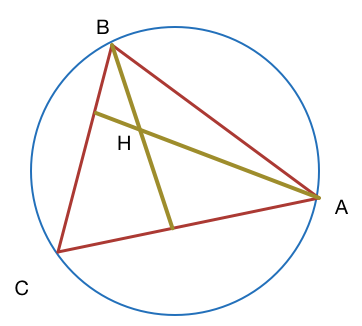# Trigonometry

Geometry Level 4$\Delta ABC$ is inscribed in a circle of radius $5$. $H$ is the orthocentre of $\Delta ABC$. If $\tan A = 1$, $\tan B = 2$ then find $CH^{2}$.

×